Select Page

## Introduction

A keyless propeller, as the name implies, requires no key for fastening the propeller on the cone of the propeller shaft. How is the torque then transferred to the propeller? The torque is transferred by the friction between the propeller and the propeller shaft.

In this article, we’ll see what important parameters govern the design of a keyless propeller, and how these can be calculated.

These are based on ABS Rules for Building and Classing Steel Vessels 2019, Part 4 Vessel systems and machinery, Section 3 Propellers. Class rules require a minimum factor of safety against the slip of 2.8, which can be used to calculate the maximum pull-up load. This load also depends on several other parameters like the type of propulsion, diameters of boss and hub, rated thrust, etc. that have been discussed below.

## Design Parameters

The following design parameters are important for the keyless propeller design:

• Minimum required mating surface pressure
• Minimum pull-up length
• Dry-fitting
• Wet-fitting
• Contact surface pressure
• Hoop stress
• Equivalent stress

Parameters like mating surface pressure and pull-up length also depend on the temperature of operation.

## Design Criteria

The design criteria as per ABS rules are as follows:

• The factor of safety against the slip of the propeller hub on the tail shaft taper at 35°C (95°F) is to be at least 2.8 under the action of maximum continuous ahead rated torque plus torque due to torsional vibrations.
• The maximum equivalent uniaxial stress (von Mises-Hencky criteria) in the hub at 0°C (32°F) is not to exceed 70% of the minimum specified yield stress or 0.2% proof stress of the propeller material.
• The calculations submitted must cover the following parameters:
• Theoretical contact surface area
• The maximum permissible pull-up length at 0°C (32°F) as limited by the maximum permissible uniaxial stress specified above
• The minimum pull-up length and contact pressure at 35°C (95°F) to attain a safety factor against slip of 2.8
• The proposed pull-up length and contact pressure at the fitting temperature
• The rated propeller ahead thrust

## Inputs

The calculations will require multiple inputs related to the propeller’s geometry and material, along with the vessel’s parameters like speed.

The inputs all follow the rule requirements by ABS, and are listed below. The diagram below also depicts some of the inputs.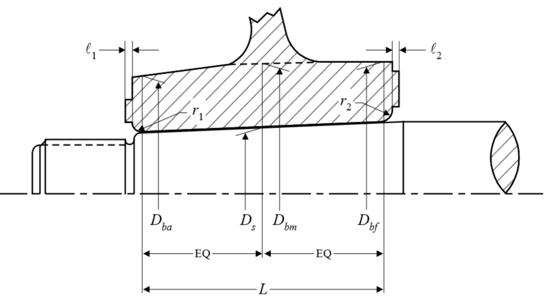• Contact surface area between propeller hub and shaft taper (A)
• Coefficient of friction between mating surfaces (µ)
• Half taper of shaft (θ)
• Factor of safety against slippage at 35°C (S)
• Coefficient for type of propulsion drive (c)
• Outer diameter of hub corresponding to Ds (Dbm)
• Outer diameter of hub corresponding to the forward point of contact (Dbf)
• Outer diameter of hub corresponding to the aft point of contact (Dba)
• Mean outer diameter of hub corresponding to Ds (Db)
• Diameter of shaft at mid-point of the taper in axial direction (Ds)
• Mean propeller pitch (P)
• Rated propeller thrust
• Calculated based on Power at rated speed (H), Vessel speed at rated power (v), and Rpm at rated speed (R), OR
• from designer (T-User)
• Modulus of elasticity of hub material (Eb)
• Poisson’s ratio of hub material (νb)
• Coefficient of linear expansion of propeller hub material (αb)
• Modulus of elasticity of shaft material (Es)
• Poisson’s ratio of shaft material (νs)
• Coefficient of linear expansion of shaft material (αs)
• Yield stress or 0.2% proof stress of propeller material (σy)
• Reference temperature as per rule (tref)
• Contact length (L)

## Calculations

The formulations for the various parameters are shown below:

• Rated propeller thrust – if the designer has this value, then the designer’s value is used, else the value from rules can be used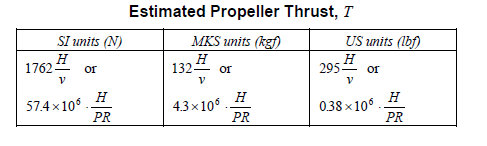• Rated Torque – Q is the rated torque which is an input corresponding to H & R, however, it can be estimated from the formula below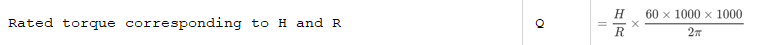• Shear force at the propeller-shaft interface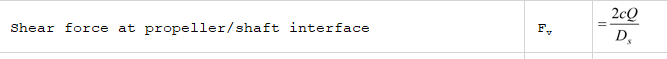• Pull-up length

The minimum pull-up length is taken at 35 degrees C, and is given by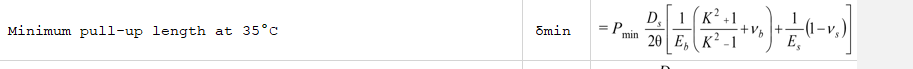The minimum pull-up length at any other temperature (less than 35 degrees) is given by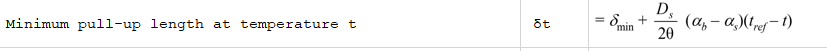• Minimum mating surface pressure at 35 degrees C is given by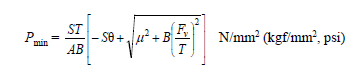The minimum mating surface pressure varies with temperature, and at another temperature less than 35 degrees C, it is given by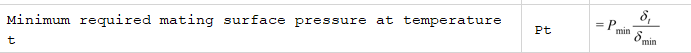Here δmin and δt are the pull-up lengths at 35 deg and at temperature ‘t’ respectively.

The pull-up load can be calculated using the formula below:

Wt (pull-up load) = A x Pt (μ + θ), where

μ = coefficient of friction between mating surfaces. For wet fitting, the friction is considerably lower than dry fitting

θ = Half taper of shaft

• Stresses: The hoop stress and equivalent stress can be given by:where K is the ratio of Db and Ds

That brings us to the end of this article. TheNavalArch also has its own product which simplifies the above calculations for the user. Please do check it out

## References

1. ABS Rules for Building and Classing Steel Vessels 2019 , Part 4 Vessel systems and machinery, Section 3 Propellers
2. https://trid.trb.org/view/63325

Disclaimer: This post is not meant to be authoritative writing on the topic presented. thenavalarch bears no responsibility for the accuracy of this article, or for any incidents/losses arising due to the use of the information in this article in any operation. It is recommended to seek professional advice before executing any activity which draws on information mentioned in this post. All the figures, drawings, and pictures are property of thenavalarch except where indicated, and may not be copied or distributed without permission.

https://thenavalarch.com/software/maritime-industry-thenavalarch/ship-design/resistance-propulsion/keyless-propeller-fitting-calculations/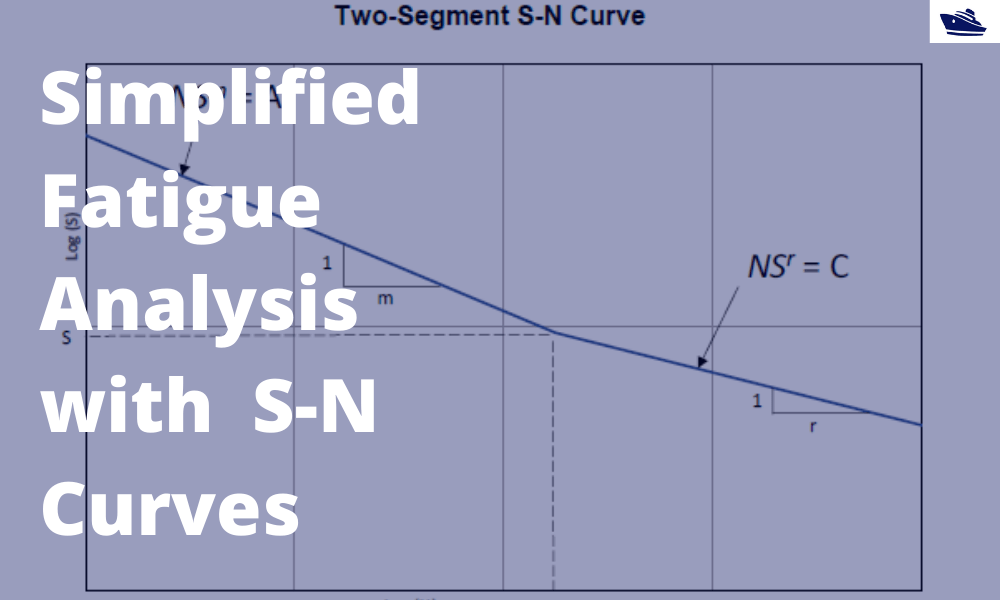## A simplified method of performing fatigue analysis of offshore structures

A simplified method of performing fatigue analysis of offshore structures   Introduction An offshore structure is subject to environmental loads of waves, wind and current. By their nature, the resulting wave loads on the structure are cyclical. These cyclical...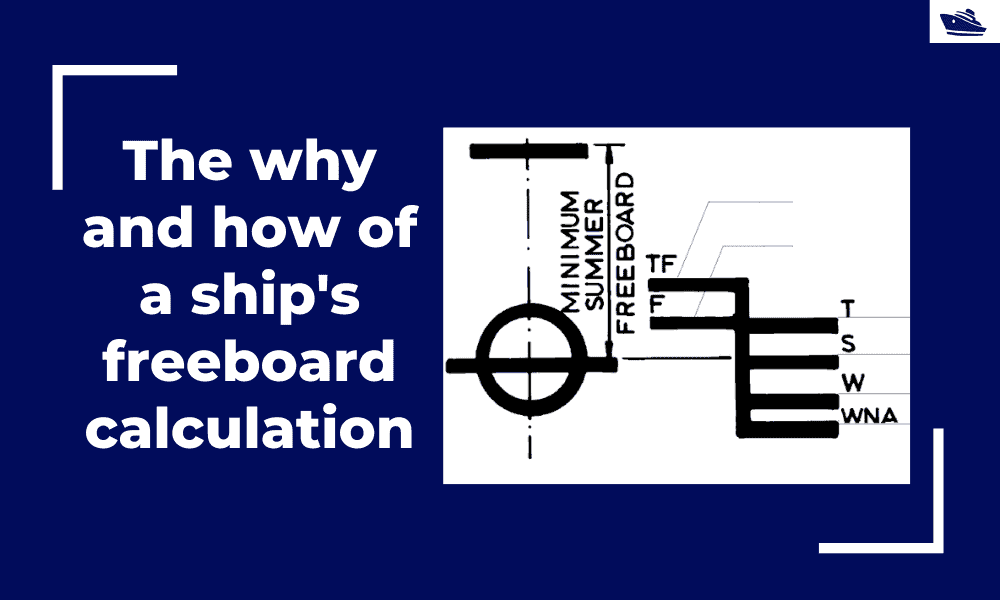## The why and how of freeboard calculation of a ship

Introduction Freeboard is a common term used in vessel operations. Freeboard is the smallest vertical distance between the waterline and the freeboard deck (generally the upper deck) along the length of the vessel. The term ‘smallest’ is of significance, as the height...

•
•
•
•
•
•